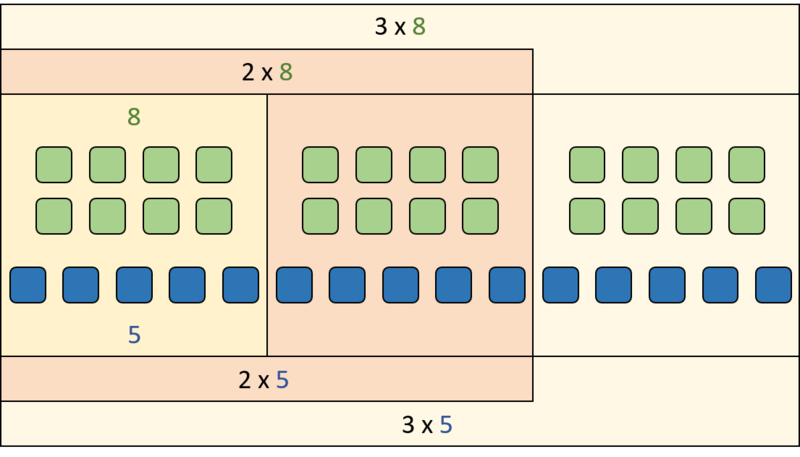# Equivalent Ratios 2

Alignments to Content Standards: 6.RP.A

In a marching band there are 24 trombones and 15 snare drums.

1. Heather says, “The ratio of trombones to snare drums is 24:15.” Audrey says, “No, the ratio of trombones to snare drums is 8:5.” Who is right, and why?

2. A different marching band has 30 snare drums, but its trombone to snare drum ratio is the same as the first marching band. How many trombones does it have?

3. Explain what these ratios all have in common:  8:5, 16:10, 24:15. Create a diagram to support your answer.

4. Mathematicians call these “equivalent ratios.” Come up with a new set of ratios that are equivalent to 6:21.

5. Explain in your own words the meaning of “equivalent ratios.”

## IM Commentary

The purpose of this task is to make explicit the meaning of equivalent ratios. Students create, analyze, and draw diagrams of two different sets of equivalent ratios, and then they write their own definition of "equivalent ratios" in their own words.

This task should not be students' first encounter with equivalent ratios, but rather, should cap off lots of work with sets of equivalent ratios in many contexts using different representations.

We suggest showing a few minutes of a performance by a large university marching band (there's an example embedded at the bottom of this commentary) before starting this task (or maybe during a short break). Students who participate in band might think some of the quantities given are unrealistic, but some marching bands are quite large. A teacher could take a few minutes to see how many instruments students can identify.

Part (a) is meant to clarify that even though 24:15 and 8:5 are written with different numbers, that they both correctly describe the situation. Using language like "there are 24 trombones for every 15 snare drums" and "there are 8 trombones for every 5 snare drums" can help reinforce this, especially with an appropriately constructed diagram. When experienced practitioners talk about "the ratio," they often automatically assume a reduced form written with the smallest possible whole numbers; but there's nothing about the mathematics that requires this.

Students who have worked with unit rates, especially if they have come to see unit rates as useful, may generate something like $\frac{8}{5}:1$ in part (c) and $\frac{2}{7}:1$ in part (d). This could present an opportunity for a nice conversation about keeping a context in mind when representing quantities (involving aspects of MP2). There's nothing mathematically wrong with saying that $\frac{8}{5}:1$ is a ratio equivalent to the others in part (c), and it may be a good use of time to point out how we can see $\frac{8}{5}:1$ in a diagram. At the same time, we can recognize that it would be impossible for a marching band to contain $\frac{8}{5}$ (a little more than one and a half) trombones.

The solution to part (e) is given in a form that a student might produce. More formally, we might say "Given a ratio $a : b$, an equivalent ratio is any ratio of the form $sa : sb$ where $s$ is a positive number." Students are likely to say "multiply or divide" instead of just "multiply." A teacher might decide to let this go or to take the time to talk about how in math we like to write definitions as simply as possible. Since, for example, dividing by 4 is the same as multiplying by $\frac{1}{4}$, we can get away with just saying "multiply."

## Solution

1. They are both right! These are different ways of correctly describing the situation.

2. Here is the given information and what we want to find organized in a ratio table:

 trombones snare drums 24 8 ? 15 5 30

In the snare drum row, I notice that 30 is 6 groups of 5. So the number of trombones indicated by the ? must be 6 groups of 8, or 48 trombones.

1. Each ratio is a multiple of 8 and 5. Here is a diagram that shows this:But drawing all those squares is cumbersome! We could choose a different representation; for example, here is a double number line diagram:1. Answers may vary. Some ratios that are equivalent to 6:21 are 2:7, 4:14, and 12:42.

2. You can get an equivalent ratio if you start with the two numbers that make the ratio and multiply them by the same number.